# How to create a matrix with random values in R?

• Last Updated : 16 May, 2021

In this article, we will discuss hw to create a matrix with random values in the R programming language.

The functions which are used to generate the random values are:

• rnorm()
• runif()
• rexp()
• rpois()
• rbinom()
• sample()

We will use all these functions one by one to create the matrix with random values.

Method 1: Using rnorm()

rnorm() function basically creates the random values according to the normal distribution.

Syntax: rnorm(n, mean, sd)

So, we give 25 as an argument in the rnorm() function, after that put those values in the matrix function with the row number and create the matrix.

## R

 `# matrix create with the help``# of matrix function random values``# generated with the help of rnorm()``m<-``matrix``(``rnorm``(25) , nrow = 5)`` ` `# print the matrix``print``(m)`

Output: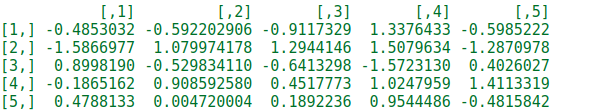Method 2: Using runif() function

runif() function basically creates the random values according to the uniform distribution. So, we give 25 as an argument in the runif() function.

Syntax: runif(n, min, max)

Parameters:
n: represents number of observations
min, max: represents lower and upper limits of the distribution

Code:

## R

 `# matrix create with the help ``# of matrix function random values ``# generated with the help of runif()``m <- ``matrix``( ``ruif``(25), nrow = 5)`` ` `# print the matrix``print``(m)`

Output: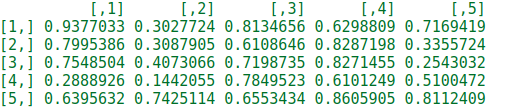Method 3: Using rexp() function

rexp() function basically creates the random values according to the exponential distribution. So, we give 25 as an argument in the rexp() function.

Syntax: rexp(N, rate )

Code:

## R

 `# matrix create with the help ``# of matrix function random values``# generated with the help of runif()``m <- ``matrix``( ``runif``(25), nrow = 5)`` ` `# print the matrix``print``(m)`

Output: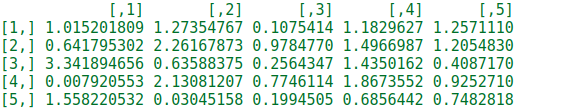Method 4: Using rpois() function

In this example, we will try to create the random values using the rpois(). rpois() function basically creates the random values according to the Poisson distribution x ~ P(lambda). So, we give 25 and 5 as an argument in the rpois() function.

Syntax: rpois(N, lambda)

Parameters:
N: Sample Size
lambda: Average number of events per interval

Code:

## R

 `# matrix create with the help ``# of matrix function random values ``# generated with the help of rpois()``m <- ``matrix``(``rpois``( 25, 5), nrow = 5)`` ` `# print the matrix``print``(m)`

Output: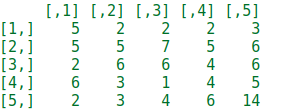Method 5: Using rbinom() function

In this example, we will try to create the random values using the rbinom(). rbinom() function basically creates the random values of a given probability.

`rbinom(n, N, p)`

Where n is the number of observations, N is the total number of trials, p is the probability of success. So, we give 25, 5, and .6 as an argument in the rbinom() function.

Code:

## R

 `# matrix create with the help``# of matrix function random values``# generated with the help of rbinom()``m <- ``matrix``(``rbinom``( 25, 5, .6), nrow = 5)`` ` `# print the matrix``print``(m)`

Output: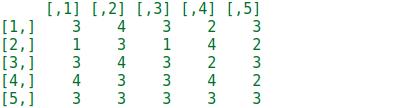Method 6: Using sample() function

In this example, we will try to create random values using the sample(). sample() function basically creates the random values of given elements.

Syntax:sample(x, size, replace)

Parameters:
x: indicates either vector or a positive integer or data frame
size: indicates size of sample to be taken
replace: indicates logical value. If TRUE, sample may have more than one same value

So, we give 1:20 and 100 as an argument in the sample() function.

Code:

## R

 `# matrix create with the help``# of matrix function random values``# generated with the help of sample()``m <- ``matrix``(``sample``(``  ``1 : 20, 100, replace = ``TRUE``), ncol = 10)`` ` `# print the matrix``print``(m)`

Output: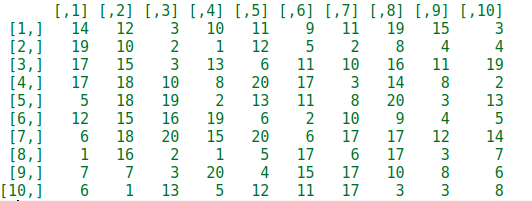My Personal Notes arrow_drop_up